Blog

# Television Minimum Signal

Also see Steps to Get TV / Signal to TV calculator.

A television minimum signal varies with model and mainly depends on the TV receiver Noise Figure (NF) and minimum Signal-to-Noise (S/N) ratio. The lower the NF and the lower the minimum S/N, the better the reception. Both NF and S/N are commonly measured on a decibels (dB) base 10 logarithmic scale (see Tech section / dB Scale).

Noise Figure
System noise figure is a measure of the noise a receiver contributes to the signal. It is the noise power ratio between input and output. Television receivers typically have a noise figure of 10 dB, but it can be as low as 6 dB, to as high as 15 dB or more.

Minimum Signal to Noise Ratio
The Signal to Noise ratio is the difference between signal power and noise power (signal / noise). A TV receiver will typically have a minimum S/N of 30 dB, some claim as low as or lower than 20 dB. In real world conditions interference from signal multi-path, other transmissions, and nearby electronic sources, effectively increases minimum S/N to 30 dB.

Summary
Specification Typical Variation
Noise Figure 10 dB 6 to 15 dB
Signal to Noise 30 dB 20 to 35 dB

Background Noise
Background noise, also called thermal or kTB noise, is based on Boltzmann's Constant constant (k), receiver noise bandwidth (B), and temperature (T). An RF channel noise bandwidth is 6 MHz. Temperature has a very minor effect, and it's standard practice to use 290°K (62°F) or 300°K (80°F) for calculations. The receiver noise floor is -106 dBm.

Signal and noise power are measured in dBm -- decibels (dB's) above or below 1 milliwatt (see Tech section / dBm Signal Power) .Published Minimum Signal
Published minimum signal is usually a measurement made under ideal conditions in a quite noise free RF shielded cage using a clean test signal. Some sources state minimum signal as -83 dBm.

Actual Minimum Signal
Most television require a minimum signal of -65 dBm, but it can be as low as -75 dBm to as high as -55 dBm. A minimum signal of -65 dBm is based on estimating real world noise interference from other transmissions and any near-by electrical and electronic sources.

Signals greater than -5 dBm will saturate (overwhelm) the receiver distorting the signal so it cannot be processed.

-65
to -5 dBm

Bars Signal (dBm) Percent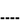Low S/N < -65 ---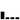Minimal -65 to -55 0 - 17%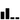Normal -55 to -45 17 - 33%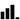Good -45 to -30 33 - 58%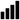Strong -30 to -5 58 - 100%

TV MINIMUM SIGNAL POWER

Most TV's and locations will
require a signal of ≥ -65 dBm.

Pmin = kTB (NF) (S/N)
 Pmin = Minimum Signal (watts). k = Boltzmann's Constant= 1.380 622 x 10-23 (Watt sec / °K). T = Temperature in Degrees Kelvin (°K). B = Receiver Noise Bandwidth in Hertz (Hz). = 6,000,000 Hz (6 MHz) for a TV RF channel. NF - Noise Figure (ratio). S/N - Minimum Signal-to-Noise (ratio).

kTB Noise (80°F)
Bandwidth Noise
1 Hz -174 dBm
6 MHz -106 dBm

 Noise Figure (NF): dB Signal-to-Noise (S/N): dB Bandwidth (B): MHz Temperature (T): °K °F °C
 Bandwidth Temperature Noise Floor (kTB) + Noise Factor (NF) = Noise out of Receiver (kTB NF) + Minimum Signal-to-Noise: Minimum TV Signal (dBm)

 OTA DTv Television Minimum Signal